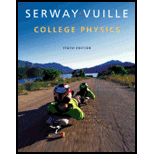# A block-spring system consists of a spring with constant k = 425 N/m attached to a 2.00-kg block on a frictionless surface. The block is pulled 8.00 cm from equilibrium and released from rest. For the resulting oscillation, find the (a) amplitude, (b) angular frequency, (c) frequency, and (d) period. What is the maximum value of the block’s (e) velocity and (f) acceleration?### College Physics

10th Edition
Raymond A. Serway + 1 other
Publisher: Cengage Learning
ISBN: 9781285737027

#### Solutions

Chapter
Section### College Physics

10th Edition
Raymond A. Serway + 1 other
Publisher: Cengage Learning
ISBN: 9781285737027
Chapter 13, Problem 17P
Textbook Problem
1311 views

## A block-spring system consists of a spring with constant k = 425 N/m attached to a 2.00-kg block on a frictionless surface. The block is pulled 8.00 cm from equilibrium and released from rest. For the resulting oscillation, find the (a) amplitude, (b) angular frequency, (c) frequency, and (d) period. What is the maximum value of the block’s (e) velocity and (f) acceleration?

a)

To determine
The amplitude of the oscillation.

### Explanation of Solution

Given info: The force constant of the spring is given 425Nm-1 . The mass of the block is 2.00 kg. The block is pulled 8.00 cm from the equilibrium position.

Explanation:

The amplitude A is the maximum distance of the object from its equilibrium position. In the absence of friction, an object in simple harmonic motion oscillates between the positions x = -A and x = +A

b)

To determine
The angular frequency of the block-spring system.

c)

To determine
The frequency of the block-spring system.

d)

To determine
The period of the oscillation.

e)

To determine
The maximum value of the block’s velocity.

f)

To determine
The maximum acceleration of the block.

### Still sussing out bartleby?

Check out a sample textbook solution.

See a sample solution

#### The Solution to Your Study Problems

Bartleby provides explanations to thousands of textbook problems written by our experts, many with advanced degrees!

Get Started

Find more solutions based on key concepts
The FDA has extensive regulatory control over supplement sad. T F

Nutrition: Concepts and Controversies - Standalone book (MindTap Course List)

The freezing point of water is _________ K.

Introductory Chemistry: A Foundation

What are the base-pairing rules for DNA? a. AG, TC b. AT, GC c. AC, TG d. AA, GG, CC, TT

Biology: The Unity and Diversity of Life (MindTap Course List)

Assume that global lightning on the Earth constitutes a constant current of 1.00 kA between the ground and an a...

Physics for Scientists and Engineers, Technology Update (No access codes included)